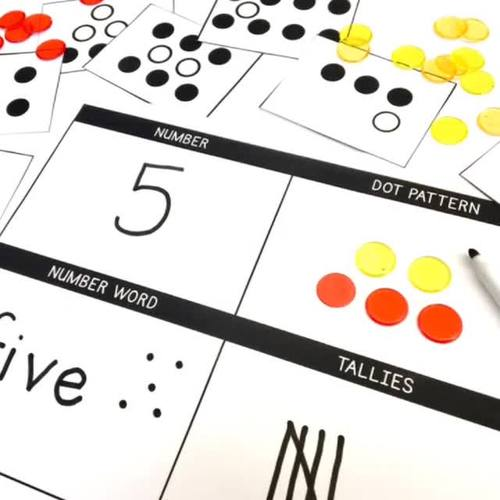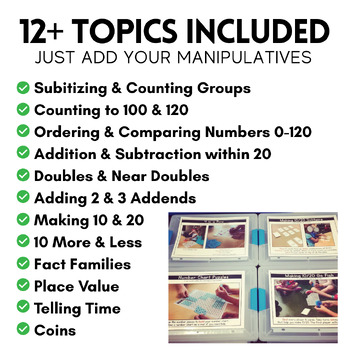# Math Centers {1st Grade} 28 Centers, Labels, and Visual DirectionsSubject
Resource Type
Format
PDF (20 MB|145 pages)
Standards
\$15.00
• Product Description
• Standards

**To see sample centers, please click on the PREVIEW button above.See this resource in action in my classroom here and here. For a 2nd grade version, click here. **

Math centers are a perfect way to review and practice spiral-review math skills. In this resource you will find 28 ready-to-print math centers, coordinating labels for your math-center bins, and visual directions.

44 Math Bin Labels

General Math Bin Labels #1-#8

One label for each individual math centers

Math Center Visual Directions & Recording Sheets

Number Sense Games

Show Me the Number

Grab and Spill

Number Chart Games

Race to 100/200

Number Chart Puzzles

4 in a Row

Pull and Compare

Number Card Games

Making 10/20 Go Fish

Making 10/20 Solitaire

Flip a Difference

Dice Games

10 More and 10 Less

Before and After

Before and After – Number Line

Find My Sum

Find My Difference

Fact Family Roll

Place Value Roll

What Can You Make?

Domino Games

Domino Doubles

Sorting Dominoes

Spinner Game

Place Value Spin

Spinner Game

Time Match

How Much Is It Worth?

Math Center Visual Directions Only (no other materials included)

Making Numbers

Math By Myself

Cuiseniare Rod Houses

Composite Shapes

Solve the Room

Hot Dots

100s Board

Show Me the Money

Versatiles

If you have any questions or requests, feel free to email me on the Q&A Section on TpT.

Catherine

The Brown-Bag Teacher

***For other teaching and learning resources, check out my Editable Classroom Newsletters and Primary Writing Prompts & Papers.

to see state-specific standards (only available in the US).
Add and subtract within 20, demonstrating fluency for addition and subtraction within 10. Use strategies such as counting on; making ten (e.g., 8 + 6 = 8 + 2 + 4 = 10 + 4 = 14); decomposing a number leading to a ten (e.g., 13 - 4 = 13 - 3 - 1 = 10 - 1 = 9); using the relationship between addition and subtraction (e.g., knowing that 8 + 4 = 12, one knows 12 - 8 = 4); and creating equivalent but easier or known sums (e.g., adding 6 + 7 by creating the known equivalent 6 + 6 + 1 = 12 + 1 = 13).
Apply properties of operations as strategies to add and subtract. If 8 + 3 = 11 is known, then 3 + 8 = 11 is also known. (Commutative property of addition.) To add 2 + 6 + 4, the second two numbers can be added to make a ten, so 2 + 6 + 4 = 2 + 10 = 12. (Associative property of addition.)
Use addition and subtraction within 20 to solve word problems involving situations of adding to, taking from, putting together, taking apart, and comparing, with unknowns in all positions, e.g., by using objects, drawings, and equations with a symbol for the unknown number to represent the problem.
Given a two-digit number, mentally find 10 more or 10 less than the number, without having to count; explain the reasoning used.
Compare two two-digit numbers based on meanings of the tens and ones digits, recording the results of comparisons with the symbols >, =, and <.
Total Pages
145 pages
N/A
Teaching Duration
1 Year
Report this Resource to TpT
Reported resources will be reviewed by our team. Report this resource to let us know if this resource violates TpT’s content guidelines.
• Ratings & Reviews
• Q & A

Teachers Pay Teachers is an online marketplace where teachers buy and sell original educational materials.